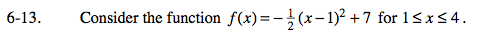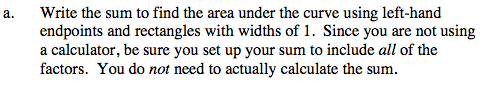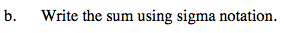### Home > PC > Chapter 6 > Lesson 6.1.1 > Problem6-13

6-13.
1. Consider the function f(x) = −(x − 1)2 + 7 for 1 ≤ x ≤ 4. Homework Help ✎

1.Write the sum to find the area under the curve using left-hand endpoints and rectangles with widths of 1. Since you are not using a calculator, be sure you set up your sum to include all of the factors. You do not need to actually calculate the sum.

2. Write the sum using sigma notation.f(1) + f(2) + f(3)$\sum_{k=1}^{3}\left(-\frac{1}{2}(??)^{2}+7\right)$